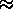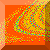# 3. Basin Boundary Structure of the Three-disk Model

Consider the configuration shown in Fig. 2. We wish to determine which exit a particle takes to leave the system when it is incident from the left at y = b and its initial direction of approach makes an anglewith respect to the y-axis. These are the two input variables which we will be varying to see how they affect the outcome of the trajectories. We define the scattering region as the area bounded by the equilateral triangle and the disks (it is shown shaded in Fig. 2). We are only interested in trajectories whose paths eventually enter the scattering region. Once a trajectory enters the scattering region, it will leave the region through one of the exits labeled in Fig. 2. (For b andrandomly chosen, there is zero probability for the trajectory to stay inside the scattering region for all time.)

To visually differentiate which initial condition leads to which exit, we have color-coded the phase space of initial conditions as follows: if a trajectory with initial condition defined by (b,) leaves through exit I (Fig. 2), then the point (b,) will be colored red. Similarly, initial conditions whose trajectories leave through exit II will be colored green, and ones that leave through exit III will be colored blue. Initial conditions whose trajectories never enter the scattering region will be colored white.

In Fig. 3 , we see pictures of the basins of attraction for the different exits at various magnification levels. From the pictures, it seems that no matter how much we amplify the magnification, there does not seem to be any regions where a boundary separates only two basins. It appears that there is always a cluster of three colors near the boundaries. This gives a clear (although nonrigorous) indication that the basin boundaries are Wada.

Before we proceed to show more rigorously that the basin boundaries for this system possess the Wada property (see Sec. 4), we shall compute the uncertainty dimension of the basin boundaries to see how tiny perturbations in the initial conditions affect the predictability of the final state. Following a method proposed by Grebogi et al. , we randomly select a large number N of initial conditions (b,) from some specified region in phase space and determine their exit modes. The exit mode calculations are now repeated for initial conditions under-perturbation of b, namely (b+,) and (b-,). If the trio of initial conditions, (b-,),(b,) and (b+,) results in trajectories that leave through the same exit, then the original initial condition (b,) is considered ``certain'' under-perturbation of b; otherwise, it is deemed ``uncertain''. For a given perturbation, we can compute the fraction f() of initial conditions which leads to uncertain final states. In general, f() scales withas f(), whereis called the uncertainty exponent. The uncertainty dimension of the basin boundaries is then defined as d = D -where D is the dimension of phase space [Grebogi et al., 1983; MacDonald et al., 1985]. In this case, D=2.

Figs. 4(b)-(d) show the log-log plots of f() vsfor three different regions in phase space as outlined in Fig. 4(a). It is seen that, for all three of these regions, the plots are well approximated by a power law, and we obtained a mean value of0.27 for the uncertainty exponent, or d1.73 for the uncertainty dimension. The implication of such a low value for the uncertainty exponent is quite striking. For example, increasing the precision in the specification of initial conditions by a factor of 10 reduces f() by only about 50% (10-0.270.54).Chaos at Maryland Home Page